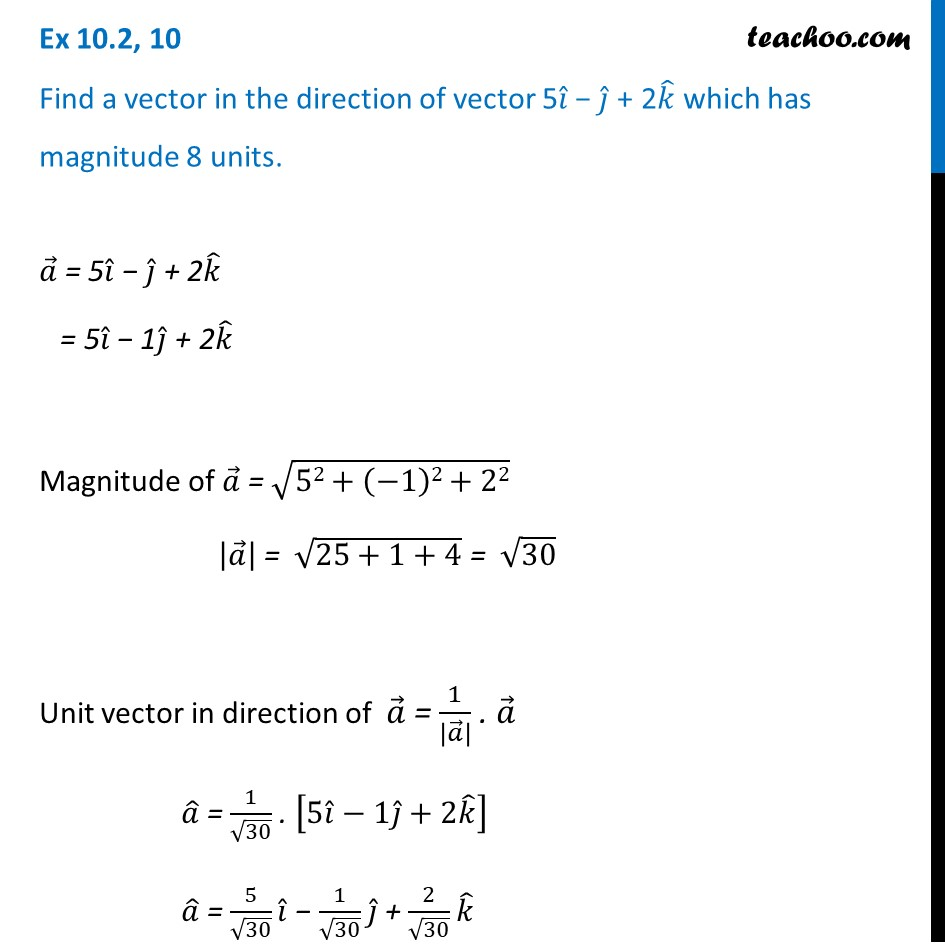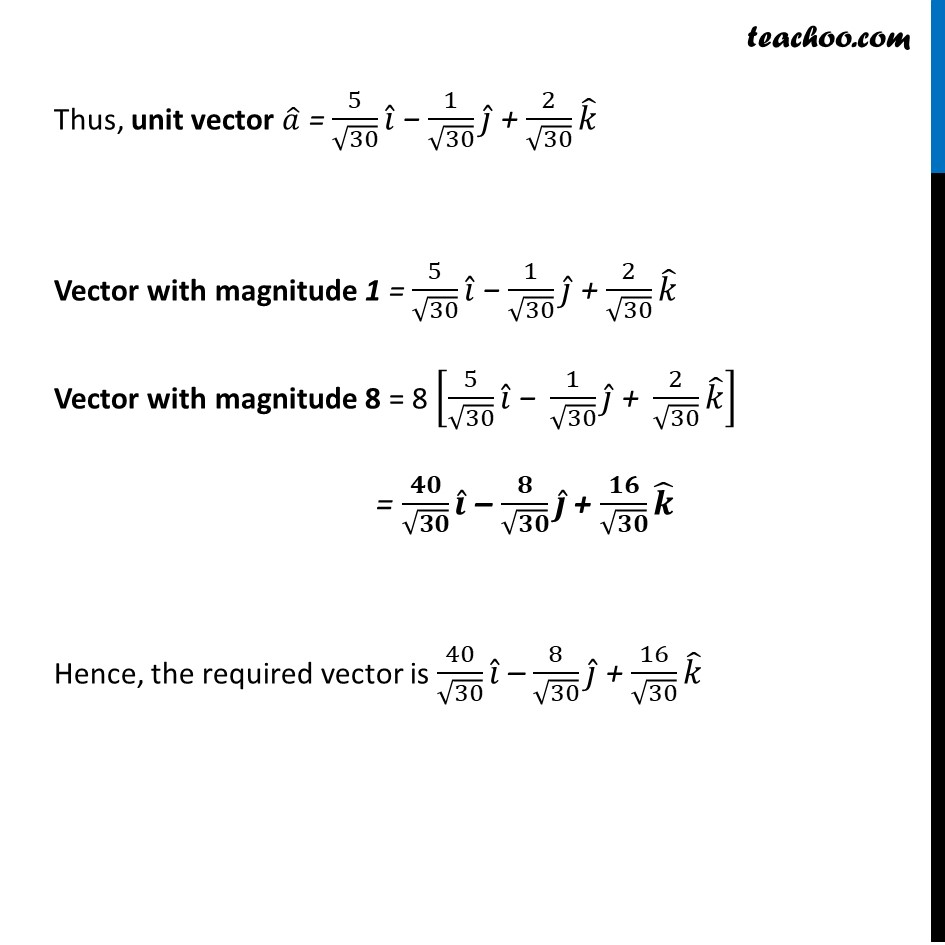1. Chapter 10 Class 12 Vector Algebra (Term 2)
2. Serial order wise
3. Ex 10.2

Transcript

Ex 10.2, 10 Find a vector in the direction of vector 5𝑖 ̂ − 𝑗 ̂ + 2𝑘 ̂ which has magnitude 8 units.𝑎 ⃗ = 5𝑖 ̂ − 𝑗 ̂ + 2𝑘 ̂ = 5𝑖 ̂ − 1𝑗 ̂ + 2𝑘 ̂ Magnitude of 𝑎 ⃗ = √(52+(−1)2+22) |𝑎 ⃗ | = √(25+1+4) = √30 Unit vector in direction of 𝑎 ⃗ = 1/|𝑎 ⃗ | . 𝑎 ⃗ 𝑎 ̂ = 1/√30 . [5𝑖 ̂−1𝑗 ̂+2𝑘 ̂ ] 𝑎 ̂ = 5/√30 𝑖 ̂ − 1/√30 𝑗 ̂ + 2/√30 𝑘 ̂ Thus, unit vector 𝑎 ̂ = 5/√30 𝑖 ̂ − 1/√30 𝑗 ̂ + 2/√30 𝑘 ̂ Vector with magnitude 1 = 5/√30 𝑖 ̂ − 1/√30 𝑗 ̂ + 2/√30 𝑘 ̂ Vector with magnitude 8 = 8 [5/√30 𝑖 ̂" − " 1/√30 𝑗 ̂" + " 2/√30 𝑘 ̂ ] = 𝟒𝟎/√𝟑𝟎 𝒊 ̂ – 𝟖/√𝟑𝟎 𝒋 ̂ + 𝟏𝟔/√𝟑𝟎 𝒌 ̂ Hence, the required vector is 40/√30 𝑖 ̂ – 8/√30 𝑗 ̂ + 16/√30 𝑘 ̂